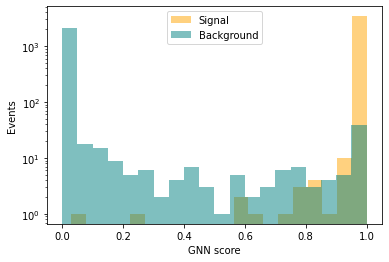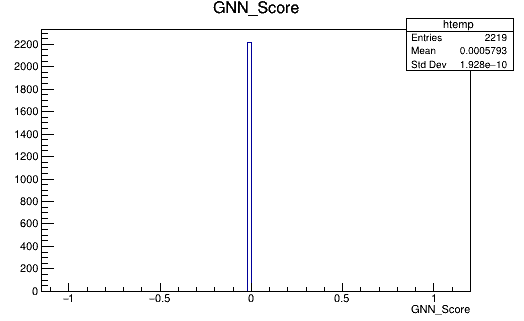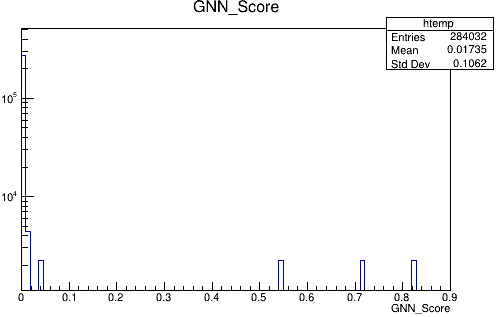# Writing np.array to a TBranch goes wrong

Dear ROOTers,

I am trying to perform a very simple task but keep getting the wrong outcome: using pyROOT to save a numpy array into a new TBranch. This is my code:

``````file = ROOT.TFile("trial.root","UPDATE")
tree = TTree("OutTree","OutTree")
json_file = open("bkg_preds.json", "r")
list_bare_output = ast.literal_eval(string_bare_output)
array_bare_output = np.array(list_bare_output)

tree.Branch("GNN_Score", array_bare_output, "GNN_Score/D")

for i in range(len(array_bare_output)):
tree.Fill()

file.Write()
file.Close()
``````

The TBranch is wrongly filled with only-zeros. I’ve noticed that when the TBranch is set e.g. as
`tree.Branch("GNN_Score", array_bare_output, "GNN_Score/D")`
the shape of the information in the branch looks “better” (but still wrong) where the nentries is clearly wrong (it is equal to 128*len(array_bare_output)).

The information being plotted should look roughly as (the background distribution):whereas upon writing to the TBranch I get:and with the `/D` option (for example) I get:How can this be achieved as simply as possible? Getting the right information and of course keeping the right number of entries?

Many thanks in advance for any help.

Roy

_ROOT Version: 6.22/06
_Platform: linuxx8664
_Compiler: gcc

Hello,

The length of the array is definitely necessary, you can see an example here (PyROOT box, Branch pythonization):

https://root.cern.ch/doc/master/classTTree.html

Also, the loop:

``````for i in range(len(array_bare_output)):
tree.Fill()
``````

will add as many events of the tree as the length of the array (which I think is not what you want), for every event the GNN_Score branch will contain exactly the same value.

The following code works:

``````import ROOT
import numpy as np

# Write tree
f = ROOT.TFile("trial.root","RECREATE")
tree = ROOT.TTree("OutTree","OutTree")
array_bare_output = np.array([1.,2.,3.])

tree.Branch("GNN_Score", array_bare_output, "GNN_Score/D")

# Fill 5 events with exactly the same value for the GNN_Score branch ([1.,2.,3.])
for i in range(5):
tree.Fill()

f.Write()
f.Close()

What you need to do is call `Branch` with the length of the array, then fill in a loop as many times as events you want in the tree, and at every iteration of that loop you might want to change the values of the elements of the array object you passed to `Branch` (otherwise the GNN_Score branch will always have the same value).